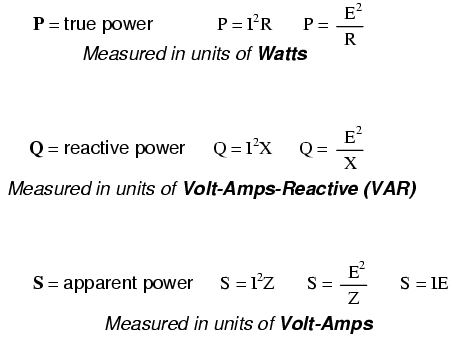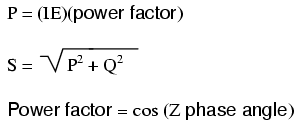# Learning Electronics

Learn to build electronic circuits

# AC circuit equations

### Inductive reactance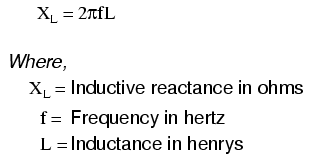### Capacitive reactance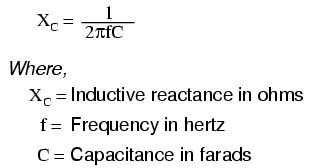ZL = R + jXL
ZC = R - jXC

### Ohm's Law for AC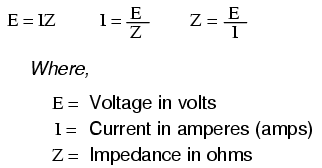### Series and Parallel Impedances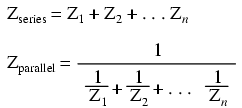NOTE: All impedances must be calculated in complex number form for these equations to work.

### Resonance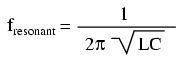NOTE: This equation applies to a non-resistive LC circuit. In circuits containing resistance as well as inductance and capacitance, this equation applies only to series configurations and to parallel configurations where R is very small.

### AC power